# Product of 455 Product of 96 Product of

• Slides: 20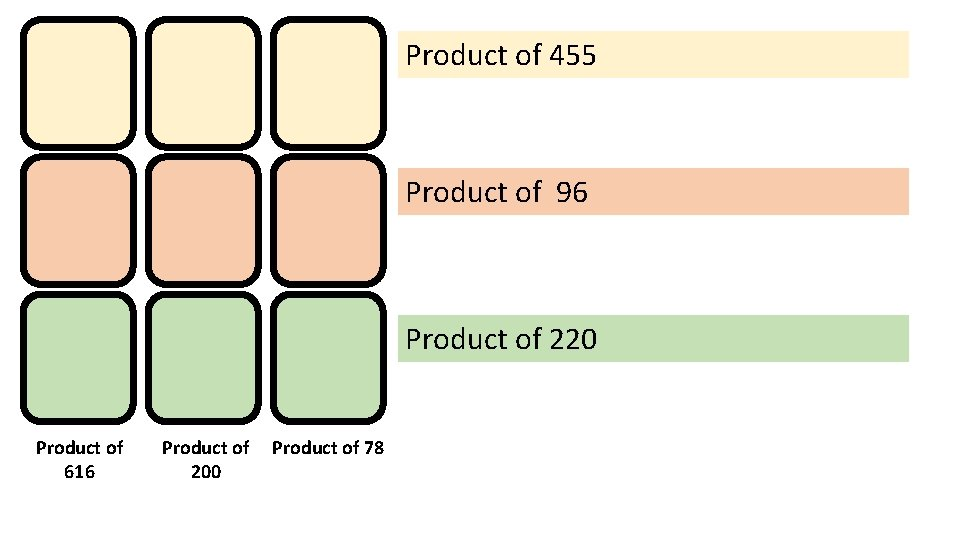Product of 455 Product of 96 Product of 220 Product of 616 Product of 200 Product of 78The numbers in this row have to add up to 12 The numbers in this row have to add up to 27 The numbers in this row have to add up to 31 The numbers in this column add to 19 to 22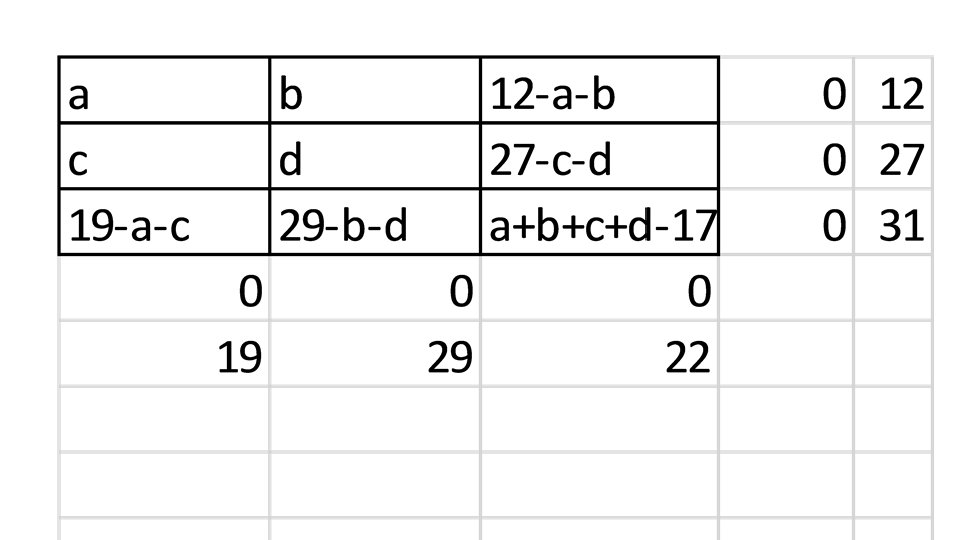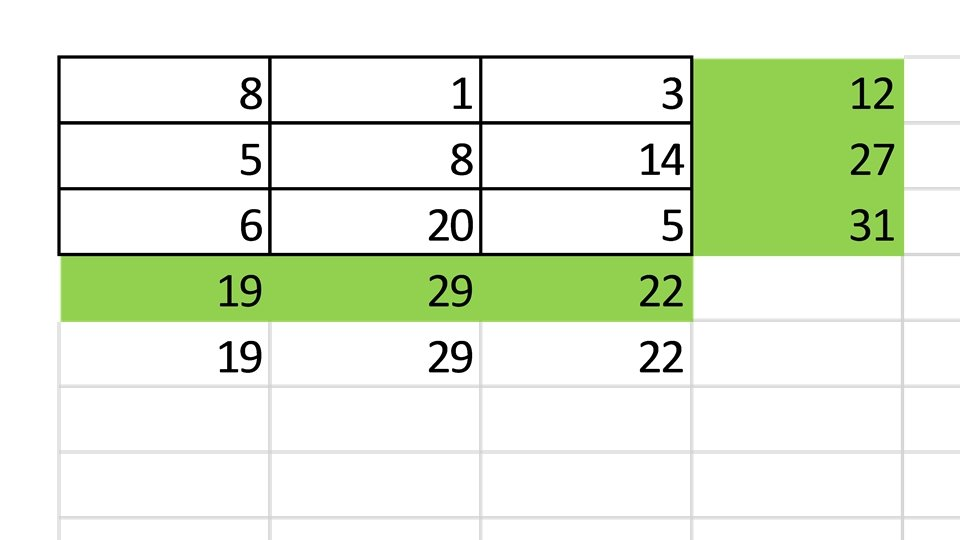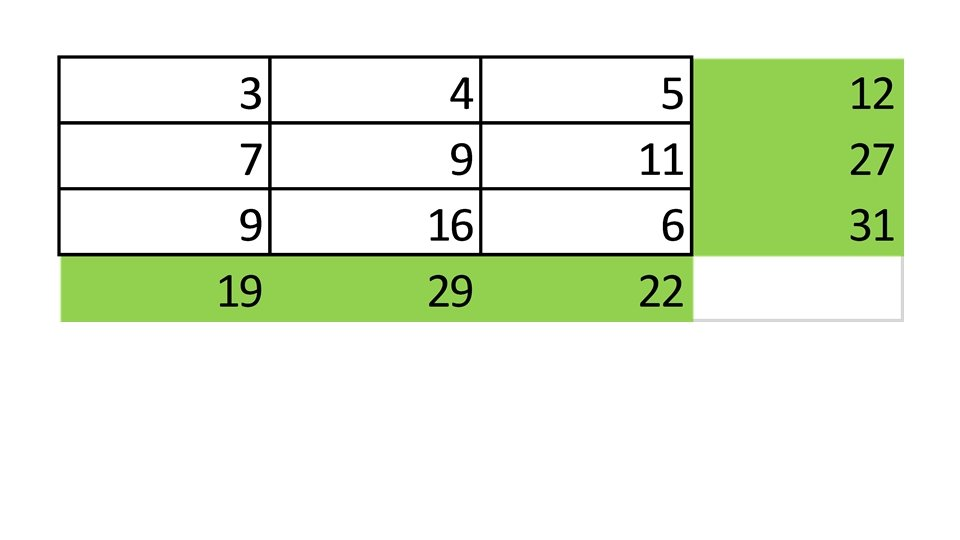7 9 3 1 11 18 4 The numbers in this row have to add up to 12 7 The numbers in this row have to add up to 27 10 The numbers in this row have to add up to 31 The numbers in this column add to 19 to 22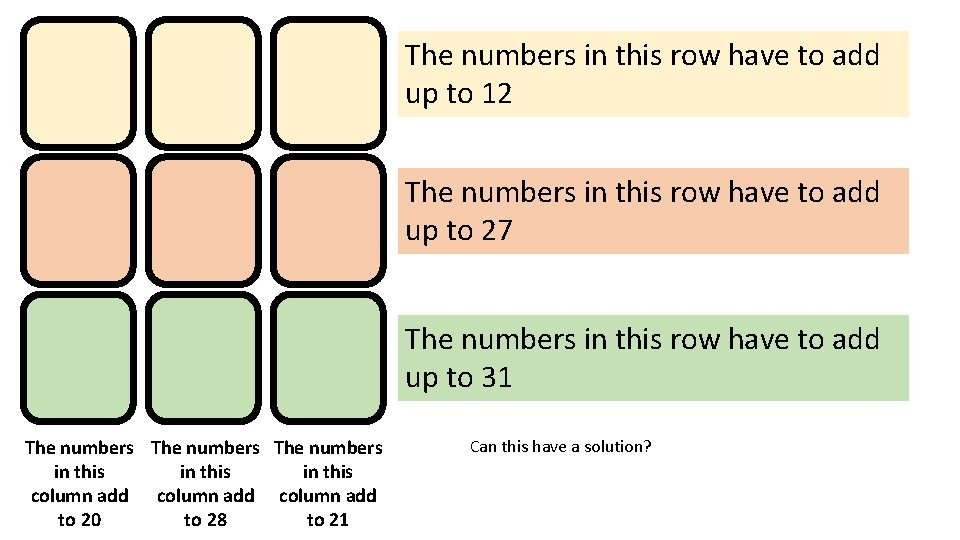The numbers in this row have to add up to 12 The numbers in this row have to add up to 27 The numbers in this row have to add up to 31 The numbers in this column add to 20 to 28 to 21 Can this have a solution?The numbers in this row are consecutive and have to add up to 18 The numbers in this row are consecutive and have to add up to 24 The numbers in this row are consecutive and have to add up to 30 The numbers in this column are consecutive odd numbers The numbers in this column are consecutive even numbers The numbers in this column are consecutive odd numbers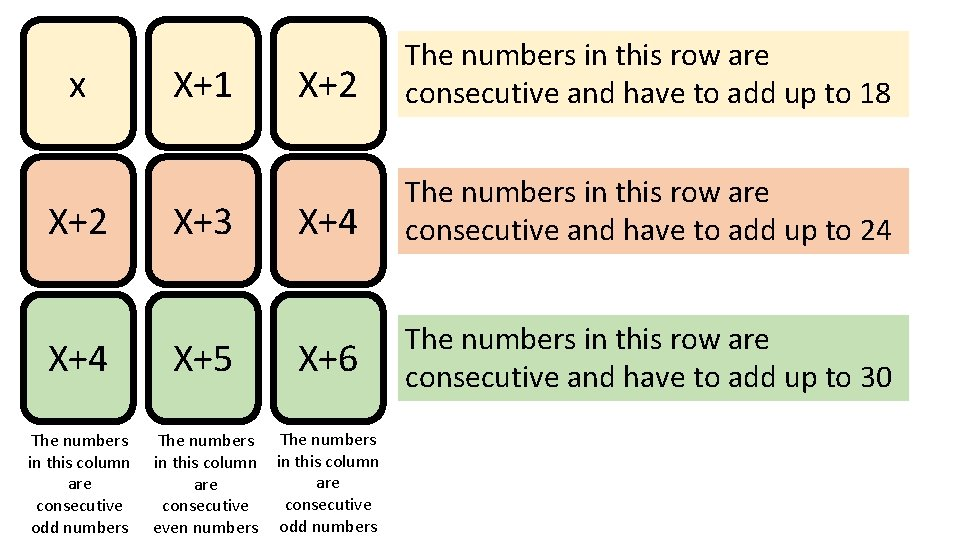x X+2 X+1 X+3 X+2 The numbers in this row are consecutive and have to add up to 18 X+4 The numbers in this row are consecutive and have to add up to 24 The numbers in this row are consecutive and have to add up to 30 X+4 X+5 X+6 The numbers in this column are consecutive odd numbers The numbers in this column are consecutive even numbers The numbers in this column are consecutive odd numbers5 7 6 8 7 The numbers in this row are consecutive and have to add up to 18 9 The numbers in this row are consecutive and have to add up to 24 The numbers in this row are consecutive and have to add up to 30 9 10 11 The numbers in this column are consecutive odd numbers The numbers in this column are consecutive even numbers The numbers in this column are consecutive odd numbersThe numbers in this row are consecutive The numbers in this column are consecutive odd numbers The numbers in this column are consecutive even numbers The numbers in this column are consecutive odd numbers11 13 12 14 13 The numbers in this row are consecutive 15 16 17 The numbers in this column are consecutive odd numbers The numbers in this column are consecutive even numbers The numbers in this column are consecutive odd numbers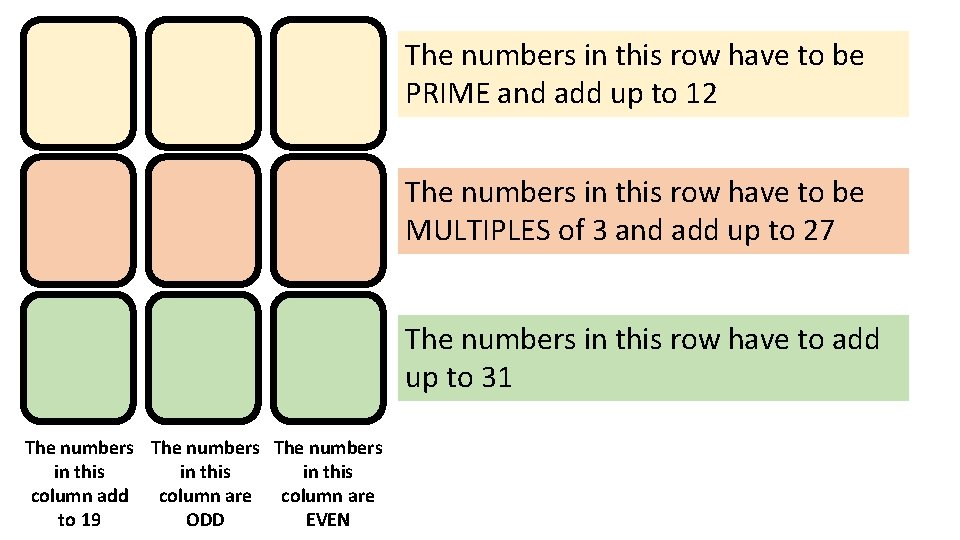The numbers in this row have to be PRIME and add up to 12 The numbers in this row have to be MULTIPLES of 3 and add up to 27 The numbers in this row have to add up to 31 The numbers in this column add column are to 19 ODD EVEN3 6 10 7 9 13 2 The numbers in this row have to be PRIME and add up to 12 12 The numbers in this row have to be MULTIPLES of 3 and add up to 27 8 The numbers in this row have to add up to 31 The numbers in this column add column are to 19 ODD EVENThe numbers in this row have to be PRIME and multiply to make 70 The numbers in this row have to be MULTIPLES of 3 & multiply to make 162 The numbers in this row have to multiply to make 32 The numbers in The numbers this column in this multiply to column are make 168 ODD EVEN7 3 8 5 9 1 2 The numbers in this row have to be PRIME and multiply to make 70 6 The numbers in this row have to be MULTIPLES of 3 & multiply to make 162 4 The numbers in this row have to multiply to make 32 The numbers in The numbers this column in this multiply to column are make 168 ODD EVEN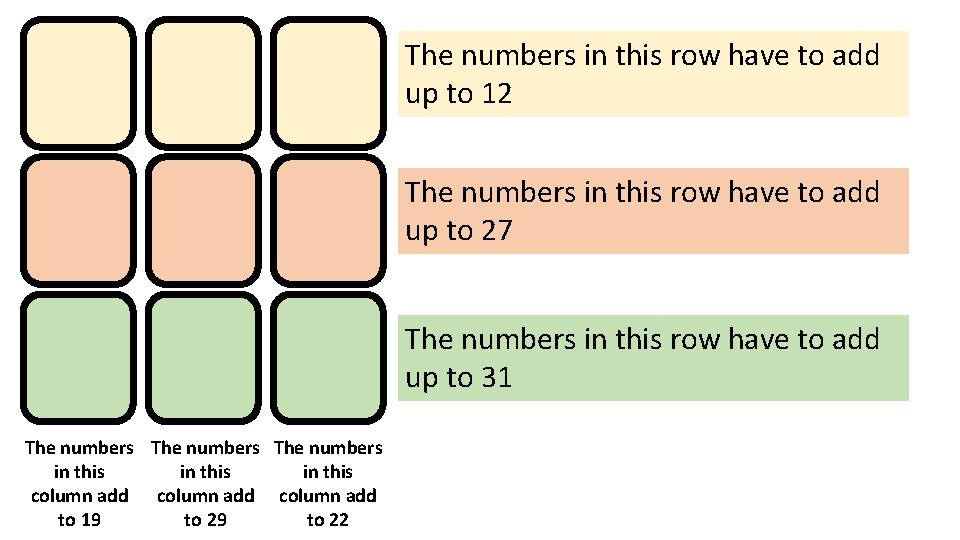The numbers in this row have to add up to 12 The numbers in this row have to add up to 27 The numbers in this row have to add up to 31 The numbers in this column add to 19 to 22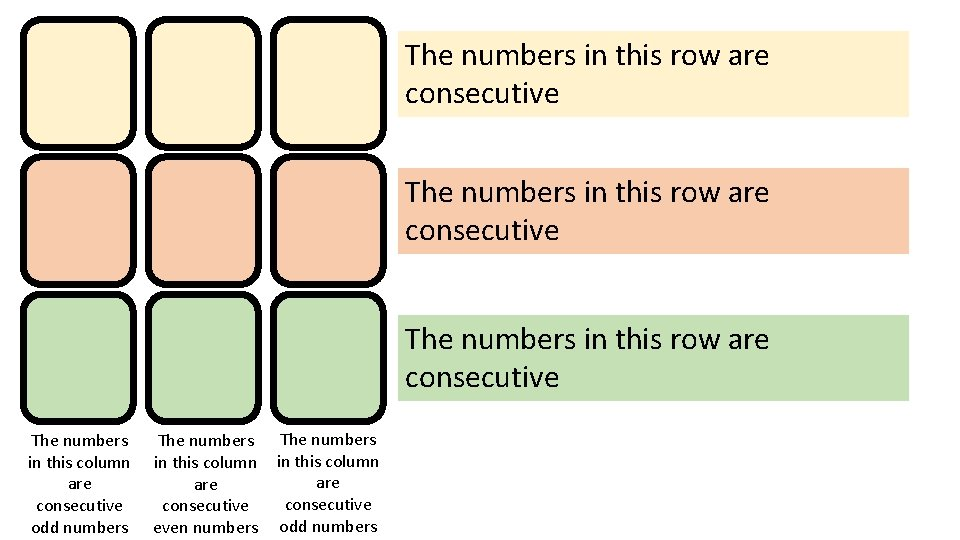The numbers in this row are consecutive The numbers in this column are consecutive odd numbers The numbers in this column are consecutive even numbers The numbers in this column are consecutive odd numbersThe numbers in this row have to be PRIME and add up to 12 The numbers in this row have to be MULTIPLES of 3 and add up to 27 The numbers in this row have to add up to 31 The numbers in this column add column are to 19 ODD EVENThe numbers in this row have to be PRIME and multiply to make 70 The numbers in this row have to be MULTIPLES of 3 & multiply to make 162 The numbers in this row have to multiply to make 32 The numbers in The numbers this column in this multiply to column are make 168 ODD EVEN